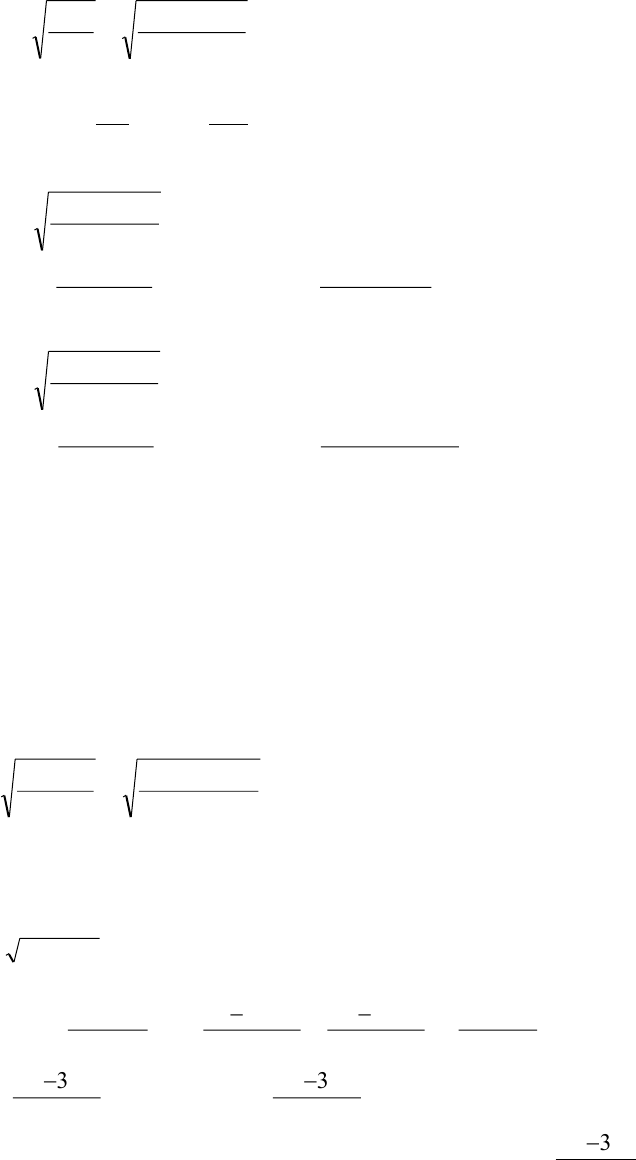Study Guides (400,000)
CA (160,000)
UTSC (10,000)
Final

# MGOC20H3 Lecture Notes - Lecture 1: Admissible Set, Operations ManagementExam

Department
Management
Course Code
MGOC20H3
Professor
Vinh Quan
Study Guide
Final

This preview shows page 1. to view the full 4 pages of the document.1
Department of Management, UTSC
MGOC20 Operations Management: A Mathematical Approach L01, Midterm Solution
Q1.
(a)
p4 = \$18.75, H = \$18.75(.10) = \$1.875/unit/year
875.1
)460)(40(22
4== H
SD
EOQ
= 140.095, EOQ4 is not admissible → set Q = 400
From
2
)( HQ
pD
Q
SD
QTC ++=
TC(400) = (40)(460)/(400) + 18.75(460) + (1.88)(400)/2 = \$9047
p3 = \$19.00, H = \$19.00(.10) = \$1.90/unit/year
90.1
)460)(40(2
3=EOQ
= 139.17, EOQ3 is not admissible → set Q = 200
TC(200) =
(40)(460) (1.90)(200)
(19.00)(460)
200 2
++
= \$9022.00
p2 = \$19.50, H = \$19.50(.10) = \$1.95/unit/year
95.1
)460)(40(2
2=EOQ
=137.37, EOQ2 is admissible so stop set Q = 137.37
TC(q2*) =
(40)(460) (1.95)(137.37)
(19.55)(460)
137.37 2
++
= \$9237.88
Therefore it is best to order 200 units each time since it has lowest cost. Total annual cost will be
\$9022.00
(b) # of orders = D/Q* = 460/200 =2.3,
# of days between orders = Q*/D = 200/460 = .434 * 365 = 158.7 days
Q2.
E[L] = (5 + 2)/2 = 3.5 weeks, VAR[L] = (5/50 2/50)2/12 = 0.0003 years
700
1
)5000)(49(2][2
*==H
DSE
Q
boxes
lead time demand X is normally dist. with mean = E[X]= E[L]* E[D] = (3.5/50)(5000) = 350
var[X] = E[L]*var[D] + E[D]2 * var[L] = (3.5/50)(45)2 + 50002(0.0003) = 7641.75
= 87.4171, CB = \$5/box/year
][
*
*][DEC
hq
rXP
B
=
,
)5000(5
)700)(1(
]
][*][
[=
XX
XErXEX
P
,
028.0]
4171.87
]350*
[=
r
ZP
,
972.0028.1]
4171.87
]350*
[==
r
ZP
From Z-Table, P[Z ≤ 1.91] = .9719 close enough, solve
4171.87
350*
91.1
=r
get r* = 516.97 boxes.